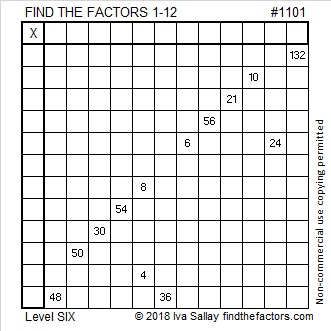# 1101 and Level 6

Which common factor of 6 and 24 will help you solve this puzzle? 2, 3, or 6?
Likewise, possible common factors of 8 and 4 are 1, 2, and 4, and for 48 and 36, you must choose between 4, 6, and 12.

In each case, only one choice will work with all the other clues in the puzzle.  You can figure out the correct choices and complete the entire puzzle by using logic. Good luck!Print the puzzles or type the solution in this excel file: 12 factors 1095-1101

Here are a few facts about the number 1101:

Since it is made with a zero and exactly three identical numbers, it is divisible by 3.

• 1101 is a composite number.
• Prime factorization: 1101 = 3 × 367
• The exponents in the prime factorization are 1 and 1. Adding one to each and multiplying we get (1 + 1)(1 + 1) = 2 × 2 = 4. Therefore 1101 has exactly 4 factors.
• Factors of 1101: 1, 3, 367, 1101
• Factor pairs: 1101 = 1 × 1101 or 3 × 367
• 1101 has no square factors that allow its square root to be simplified. √1101 ≈ 33.181321101 is a palindrome when it is written in two other bases:
It’s 373 in BASE 18 because 3(18²) + 7(18) + 3(1) = 1101,
and 1J1 in BASE 25 (J is 19 in base 10) because 25² + 19(25) + 1 = 1101